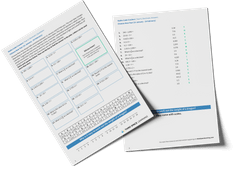Math teaching support you can trustone-on-one tutoring sessionsschools supported

[FREE] Fun Math Games & Activities Packs

Always on the lookout for fun math games and activities in the classroom? Try our ready-to-go printable packs for students to complete independently or with a partner!

# Bar Model Multiplication And Division: How To Teach Multiplication And Division Using The Bar Model

Bar model multiplication is an effective method for teaching multiplication in elementary school. As student’s progress towards middle school, it’s important to instill a deep understanding of the concept of multiplication as repeated addition, rather than rote memorization of multiplication tables: bar model multiplication is a great way to do this.

### What is bar model multiplication and division?

What is a bar model? Bar modelling is a math mastery technique and part of the Singapore math framework. Bar model multiplication and division bars utilize the bar model method, visual representation and the concrete pictorial abstract approach to illustrate the concept of multiplication and division.

Bar models, also known as strip diagrams, are similar to a number line and can also be used to teach math concepts such as fractions, using a fraction bar model, percentages, place value, decimals, addition, subtraction and can be helpful for solving word problems and number sentences.

### Why use bar model multiplication and division

Children begin learning simple multiplication facts and division in first and second grade, increasing their knowledge of multiplication tables in 3rd grade and beyond.

The concept of multiplication is typically introduced by adding the same number together multiple times or skip counting. By using a bar model, teachers can demonstrate that one whole can be divided into equal, smaller parts.

Teachers can draw bar models to demonstrate this:

By drawing a bar model and separating the bar into equal parts, children can visualize the process of multiplication and division and how they have an inverse relationship.

### Bar models and problem solving

Bar models are particularly useful when it comes to solving bar model word problems such as one-step multiplication word problems, two-step word problems and multi-step math problems. Bar models enable students to extract the most important information to solve number problems.

Third Space Learning often incorporates bar modeling into our online one-to-one tuition lessons. Paying close attention to each individual student, Third Space Learning identifies their learning gaps and moulds the program to their needs.

#### Bar model multiplication in action

Here is an example of a multiplication word problem and how it can be solved using bar model multiplication:

Mary has 26 flowers, John has 3 times the amount of flowers as Mary and Jane has 14 more flowers than John. How many flowers does Jane have?

In this example, the bar model is helpful in identifying the missing number. By presenting the problem pictorially, students can more easily understand the steps they need to take to solve the problem.

#### Example of bar model division

Here is an example of a division word problem and how it can be solved using a bar model.

Tony spends 56 hours working each week. How many hours a day on average is Tony working?

This kind of part whole model depiction can help students understand what division really means by demonstrating that division is the act of breaking up numbers into equal groups.

Do you have students who need extra support in math?
Give your students more opportunities to consolidate learning and practice skills through personalized math tutoring with their own dedicated online math tutor.

Each student receives differentiated instruction designed to close their individual learning gaps, and scaffolded learning ensures every student learns at the right pace. Lessons are aligned with your state’s standards and assessments, plus you’ll receive regular reports every step of the way.

Personalized one-on-one math tutoring programs are available for:

The content in this article was originally written by a member of the content team Vanessa Sipple-Asher and has since been revised and adapted for US schools by elementary math teacher Katie Keeton.##### Vanessa Sipple-Asher
Third Space Learning
Content Team
Vanessa edits a lot of the content that you see on the Third Space Learning blog and once in a while, she gets the chance to write articles about maths education and how Third Space Learning can help students and teachers.
xx

#### [FREE] Fun Math Games & Activities Packs

Individual packs containing fun math games and activities to complete in the classroom.

The activities are designed to be fun, flexible and suitable for a range of abilities.